# System of equations + functions - math problems

#### Number of problems found: 7

• Line intersect segment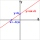Decide whether the line p : x + 2 y - 7 = 0 intersects the line segment given by points A[1, 1] and B[5, 3]
• Two gardens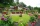The total area of the two gardens is 864 m2. The first garden is 60 m2 smaller than three times the second garden. What is the area of each garden?
• Linear function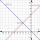What is the equation of linear function passing through points: a) A (0,3), B (3,0) b) A (-2,-6), B (3,4)Write the equation of the quadratic function which includes points A (-1, 10), B (2, 19), C (1,4)
• Car rent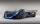Rental cars are paid a fixed daily fee plus 16 cents for each kilometer traveled. Samuel want to rent a car for a day and worked out that will pay 175 euros. When paying he got a 20% discount on the daily fee, the cost per kilometer is unchanged. Samuel t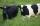Grass grows in the meadow equally fast and evenly. It is known that 99 cows graze meadow for 14 days and 95 cows by 22 days. How many cows graze meadow for 77 days?
• Function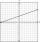For linear function f(x) = ax + ​​b ‬ is f(14)=179; f(15)=154. Calculate m, if f(m) = 2019 .

We apologize, but in this category are not a lot of examples.
Do you have an interesting mathematical word problem that you can't solve it? Submit a math problem, and we can try to solve it.

We will send a solution to your e-mail address. Solved examples are also published here. Please enter the e-mail correctly and check whether you don't have a full mailbox.

Please do not submit problems from current active competitions such as Mathematical Olympiad, correspondence seminars etc...